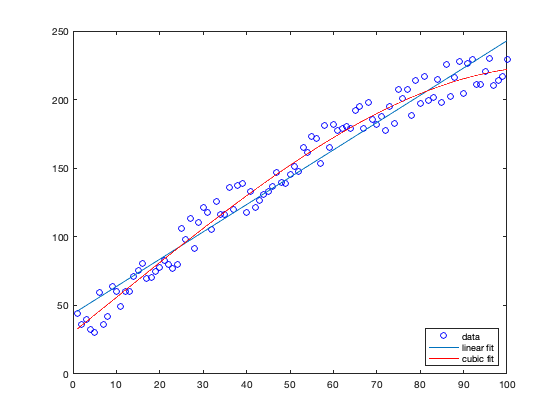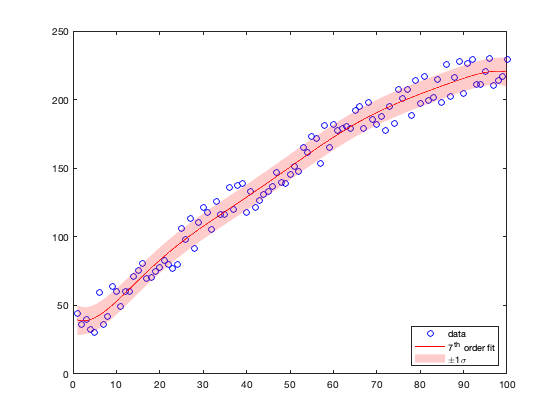# polyplot documentation

This function plots a polynomial fit to scattered x,y data. This function can be used to easily add a linear trend line or other polynomial fit to a data plot.

## Syntax

polyplot(x,y)
polyplot(x,y,n)
polyplot(...,'Name',Value,...)
polyplot(...,'error')
[h,p,delta] = polyplot(...)

## Description

polyplot(x,y) places a least-squares linear trend line through scattered x,y data.

polyplot(x,y,n) specifies the degree n of the polynomial fit to the x,y data. Default n is 1.

polyplot(...,'Name',Value,...) formats linestyle using LineSpec property name-value pairs (e.g., 'linewidth',3). If 'error' bounds are plotted, only boundedline properties are accepted.

polyplot(...,'error') includes lines corresponding to approximately +/- 1 standard deviation of errors delta. At least 50% of data should lie within the bounds of error lines. Error bounds are plotted with boundedline.

h = polyplot(...) returns handle(s) h of plotted objects.

## Examples

Given some data:

x = 1:100;
y = 12 - 0.01*x.^2 + 3*x + sind(x) + 30*rand(size(x));


Plot the data and add a simple linear trend line:

plot(x,y,'bo')
hold on
polyplot(x,y);

legend('data','linear fit','location','southeast')Instead of a linear trend, make it a cubic fit:

polyplot(x,y,3,'r');
legend('data','linear fit','cubic fit','location','southeast')Add a fat, black 7th-order polynomial fit:

polyplot(x,y,7,'k','linewidth',4);
legend('data','linear fit','cubic fit',...
'7^{th} order fit','location','southeast')Same as above, but with +/- 1 standard deviation of error lines:

figure
h(1) = plot(x,y,'bo');
hold on
h(2:3) = polyplot(x,y,7,'r','error','alpha');
legend(h,'data','7^{th} order fit','\pm1\sigma','location','southeast')## Author Info

The polyplot function and supporting documentation were created by Chad A. Greene of the University of Texas at Austin's Institute for Geophysics (UTIG). January 2015. Adapted for CDT in 2019.